Chapter 9. Chemical Bonding and Lewis Structures

# 9.5 Covalent Bonds and Lewis Structures

### Learning Objectives

By the end of this section, you will be able to:

• Define covalent bond.
• Illustrate covalent bond formation with Lewis electron dot diagrams.
• Draw Lewis structures depicting the bonding in simple molecules

Ionic bonding typically occurs when it is easy for one atom to lose one or more electrons and another atom to gain one or more electrons. However, some atoms won’t give up or gain electrons easily. Yet they still participate in compound formation. How?

There is another mechanism for obtaining a complete valence shell: sharing electrons. When electrons are shared between two atoms, they make a bond called a covalent bond.

Let us illustrate a covalent bond by using H atoms, with the understanding that H atoms need only two electrons to fill the 1s subshell. Each H atom starts with a single electron in its valence shell: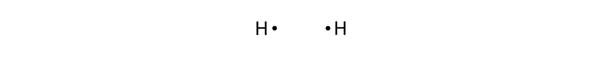The two H atoms can share their electrons: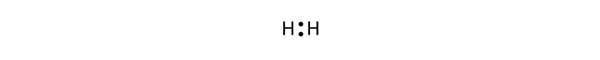We can use circles to show that each H atom has two electrons around the nucleus, completely filling each atom’s valence shell:Because each H atom has a filled valence shell, this bond is stable, and we have made a diatomic hydrogen molecule. (This explains why hydrogen is one of the diatomic elements.) For simplicity’s sake, it is not unusual to represent the covalent bond with a dash, instead of with two dots:

H–H

Because two atoms are sharing one pair of electrons, this covalent bond is called a single bond.

As another example, consider fluorine. F atoms have seven electrons in their valence shell: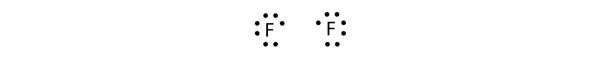These two atoms can do the same thing that the H atoms did; they share their unpaired electrons to make a covalent bond.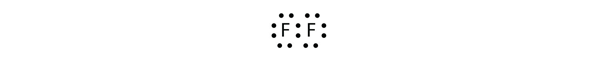Note that each F atom has a complete octet around it now:We can also write this using a dash to represent the shared electron pair: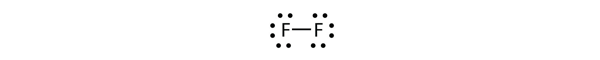There are two different types of electrons in the fluorine diatomic molecule. The bonding electron pair makes the covalent bond. Each F atom has three other pairs of electrons that do not participate in the bonding; they are called lone electron pairs. Each F atom has one bonding pair and three lone pairs of electrons.

Covalent bonds can be made between different elements as well. One example is HF. Each atom starts out with an odd number of electrons in its valence shell: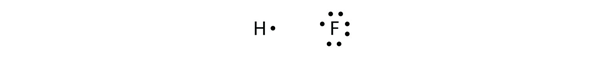The two atoms can share their unpaired electrons to make a covalent bond: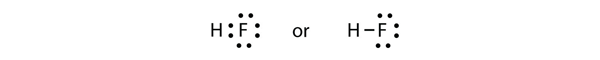We note that the H atom has a full valence shell with two electrons, while the F atom has a complete octet of electrons.

### Example 1

Use Lewis electron dot diagrams to illustrate the covalent bond formation in HBr.

Solution

HBr is very similar to HF, except that it has Br instead of F. The atoms are as follows: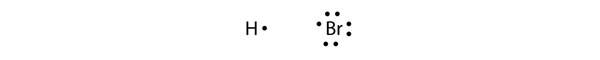The two atoms can share their unpaired electron: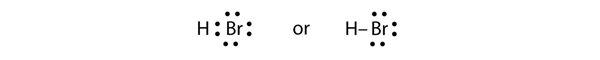Test Yourself

Use Lewis electron dot diagrams to illustrate the covalent bond formation in Cl2.

More than two atoms can participate in covalent bonding, although any given covalent bond will be between two atoms only. Consider H and O atoms: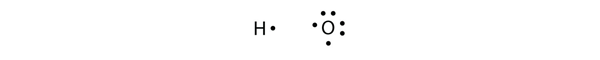The H and O atoms can share an electron to form a covalent bond: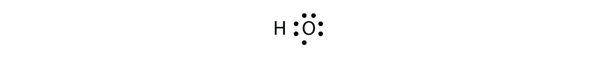The H atom has a complete valence shell. However, the O atom has only seven electrons around it, which is not a complete octet. We fix this by including a second H atom, whose single electron will make a second covalent bond with the O atom:(It does not matter on what side the second H atom is positioned.) Now the O atom has a complete octet around it, and each H atom has two electrons, filling its valence shell. This is how a water molecule, H2O, is made.

### Example 2

Use a Lewis electron dot diagram to show the covalent bonding in NH3.

Solution

The N atom has the following Lewis electron dot diagram: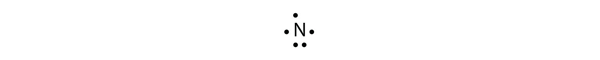It has three unpaired electrons, each of which can make a covalent bond by sharing electrons with an H atom. The electron dot diagram of NH3 is as follows:

## Lewis Structures

We also use Lewis symbols to indicate the formation of covalent bonds, which are shown in Lewis structures, drawings that describe the bonding in molecules and polyatomic ions. For example, when two chlorine atoms form a chlorine molecule, they share one pair of electrons: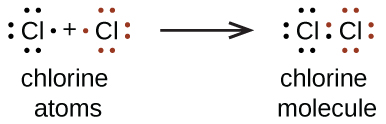The Lewis structure indicates that each Cl atom has three pairs of electrons that are not used in bonding (called lone pairs) and one shared pair of electrons (written between the atoms). A dash (or line) is sometimes used to indicate a shared pair of electrons: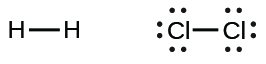A single shared pair of electrons is called a single bond. Each Cl atom interacts with eight valence electrons: the six in the lone pairs and the two in the single bond.

## The Octet Rule

The other halogen molecules (F2, Br2, I2, and At2) form bonds like those in the chlorine molecule: one single bond between atoms and three lone pairs of electrons per atom. This allows each halogen atom to have a noble gas electron configuration. The tendency of main group atoms to form enough bonds to obtain eight valence electrons is known as the octet rule.

The number of bonds that an atom can form can often be predicted from the number of electrons needed to reach an octet (eight valence electrons); this is especially true of the nonmetals of the second period of the periodic table (C, N, O, and F). For example, each atom of a group 14 element has four electrons in its outermost shell and therefore requires four more electrons to reach an octet. These four electrons can be gained by forming four covalent bonds, as illustrated here for carbon in CCl4 (carbon tetrachloride) and silicon in SiH4 (silane). Because hydrogen only needs two electrons to fill its valence shell, it is an exception to the octet rule. The transition elements and inner transition elements also do not follow the octet rule: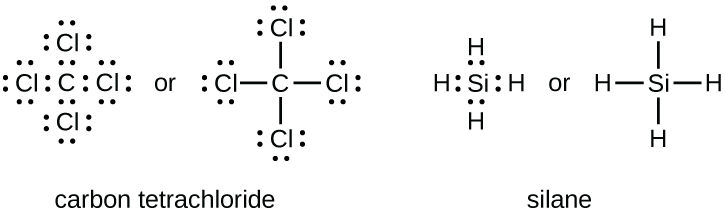Group 15 elements such as nitrogen have five valence electrons in the atomic Lewis symbol: one lone pair and three unpaired electrons. To obtain an octet, these atoms form three covalent bonds, as in NH3 (ammonia). Oxygen and other atoms in group 16 obtain an octet by forming two covalent bonds: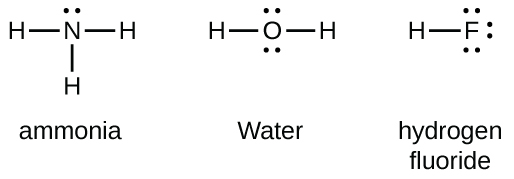## Double and Triple Bonds

As previously mentioned, when a pair of atoms shares one pair of electrons, we call this a single bond. However, a pair of atoms may need to share more than one pair of electrons in order to achieve the requisite octet. A double bond forms when two pairs of electrons are shared between a pair of atoms, as between the carbon and oxygen atoms in CH2O (formaldehyde) and between the two carbon atoms in C2H4 (ethylene):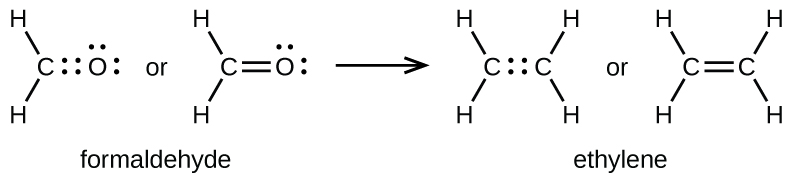A triple bond forms when three electron pairs are shared by a pair of atoms, as in carbon monoxide (CO) and the cyanide ion (CN):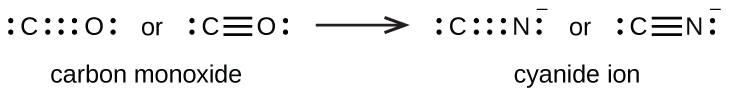## Writing Lewis Structures with the Octet Rule

For very simple molecules and molecular ions, we can write the Lewis structures by merely pairing up the unpaired electrons on the constituent atoms. See these examples: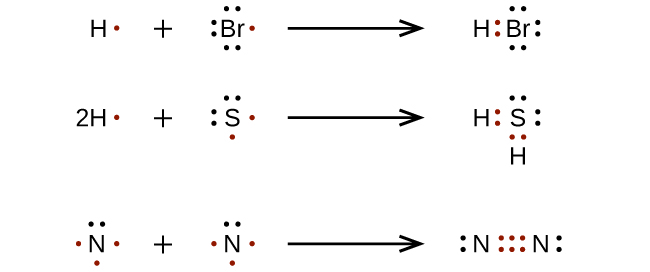For more complicated molecules and molecular ions, it is helpful to follow the step-by-step procedure outlined here:

1. Determine the total number of valence (outer shell) electrons. For cations, subtract one electron for each positive charge. For anions, add one electron for each negative charge.
2. Draw a skeleton structure of the molecule or ion, arranging the atoms around a central atom. (Generally, the least electronegative element should be placed in the center.) Connect each atom to the central atom with a single bond (one electron pair).
3. Distribute the remaining electrons as lone pairs on the terminal atoms (except hydrogen), completing an octet around each atom.
4. Place all remaining electrons on the central atom.
5. Rearrange the electrons of the outer atoms to make multiple bonds with the central atom in order to obtain octets wherever possible.

Let us determine the Lewis structures of SiH4, CHO2−, NO+, and OF2 as examples in following this procedure:

1. Determine the total number of valence (outer shell) electrons in the molecule or ion.
• For a molecule, we add the number of valence electrons on each atom in the molecule:
$\begin{array}{r r l} \text{SiH}_4 & & \\[1em] & \text{Si: 4 valence electrons/atom} \times 1 \;\text{atom} & = 4 \\[1em] \rule[-0.5ex]{21em}{0.1ex}\hspace{-21em} + & \text{H: 1 valence electron/atom} \times 4 \;\text{atoms} & = 4 \\[1em] & & = 8 \;\text{valence electrons} \end{array}$
• For a negative ion, such as CHO2, we add the number of valence electrons on the atoms to the number of negative charges on the ion (one electron is gained for each single negative charge):
$\begin{array}{r r l} {\text{CHO}_2}^{-} & & \\[1em] & \text{C: 4 valence electrons/atom} \times 1 \;\text{atom} & = 4 \\[1em] & \text{H: 1 valence electron/atom} \times 1 \;\text{atom} & = 1 \\[1em] & \text{O: 6 valence electrons/atom} \times 2 \;\text{atoms} & = 12 \\[1em] \rule[-0.5ex]{21.5em}{0.1ex}\hspace{-21.5em} + & 1\;\text{additional electron} & = 1 \\[1em] & & = 18 \;\text{valence electrons} \end{array}$
• For a positive ion, such as NO+, we add the number of valence electrons on the atoms in the ion and then subtract the number of positive charges on the ion (one electron is lost for each single positive charge) from the total number of valence electrons:
$\begin{array}{r r l} \text{NO}^{+} & & \\[1em] & \text{N: 5 valence electrons/atom} \times 1 \;\text{atom} & = 5 \\[1em] & \text{O: 6 valence electrons/atom} \times 1 \;\text{atom} & = 6 \\[1em] \rule[-0.5ex]{21em}{0.1ex}\hspace{-21em} + & -1 \;\text{electron (positive charge)} & = -1 \\[1em] & & = 10 \;\text{valence electrons} \end{array}$
• Since OF2 is a neutral molecule, we simply add the number of valence electrons:
$\begin{array}{r r l} \text{OF}_{2} & & \\[1em] & \text{O: 6 valence electrons/atom} \times 1 \;\text{atom} & = 6 \\[1em] \rule[-0.5ex]{21em}{0.1ex}\hspace{-21em} + & \text{F: 7 valence electrons/atom} \times 2 \;\text{atoms} & = 14 \\[1em] & & = 20 \;\text{valence electrons} \end{array}$
2. Draw a skeleton structure of the molecule or ion, arranging the atoms around a central atom and connecting each atom to the central atom with a single (one electron pair) bond. (Note that we denote ions with brackets around the structure, indicating the charge outside the brackets:)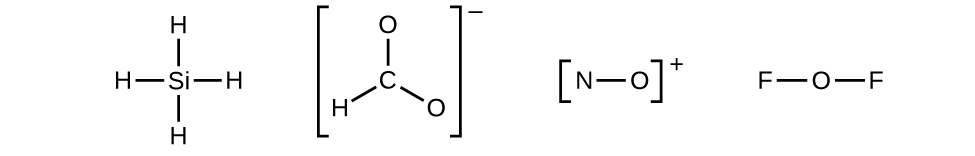When several arrangements of atoms are possible, as for CHO2, we must use experimental evidence to choose the correct one. In general, the less electronegative elements are more likely to be central atoms. In CHO2, the less electronegative carbon atom occupies the central position with the oxygen and hydrogen atoms surrounding it. Other examples include P in POCl3, S in SO2, and Cl in ClO4. An exception is that hydrogen is almost never a central atom. As the most electronegative element, fluorine also cannot be a central atom.
3. Distribute the remaining electrons as lone pairs on the terminal atoms (except hydrogen) to complete their valence shells with an octet of electrons.
• There are no remaining electrons on SiH4, so it is unchanged: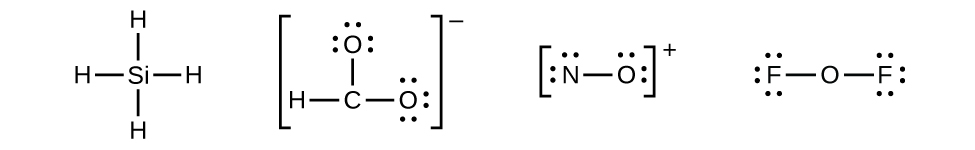4. Place all remaining electrons on the central atom.
• For SiH4, CHO2, and NO+, there are no remaining electrons; we already placed all of the electrons determined in Step 1.
• For OF2, we had 16 electrons remaining in Step 3, and we placed 12, leaving 4 to be placed on the central atom: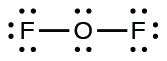5. Rearrange the electrons of the outer atoms to make multiple bonds with the central atom in order to obtain octets wherever possible.
• SiH4: Si already has an octet, so nothing needs to be done.
• CHO2: We have distributed the valence electrons as lone pairs on the oxygen atoms, but the carbon atom lacks an octet: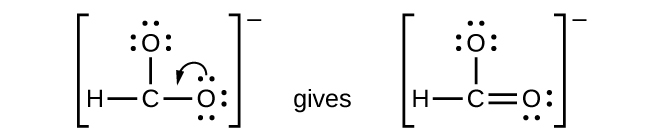• NO+: For this ion, we added eight valence electrons, but neither atom has an octet. We cannot add any more electrons since we have already used the total that we found in Step 1, so we must move electrons to form a multiple bond:This still does not produce an octet, so we must move another pair, forming a triple bond:• In OF2, each atom has an octet as drawn, so nothing changes.

Polyatomic ions are bonded together with covalent bonds, as seen in the example of CHO2−.  Because they are ions, however, they participate in ionic bonding with other ions. So both major types of bonding can occur at the same time.

### Example 3

NASA’s Cassini-Huygens mission detected a large cloud of toxic hydrogen cyanide (HCN) on Titan, one of Saturn’s moons. Titan also contains ethane (H3CCH3), acetylene (HCCH), and ammonia (NH3). What are the Lewis structures of these molecules?

Solution

1. Calculate the number of valence electrons.HCN: (1 × 1) + (4 × 1) + (5 × 1) = 10H3CCH3: (1 × 3) + (2 × 4) + (1 × 3) = 14HCCH: (1 × 1) + (2 × 4) + (1 × 1) = 10NH3: (5 × 1) + (3 × 1) = 8
2. Draw a skeleton and connect the atoms with single bonds. Remember that H is never a central atom: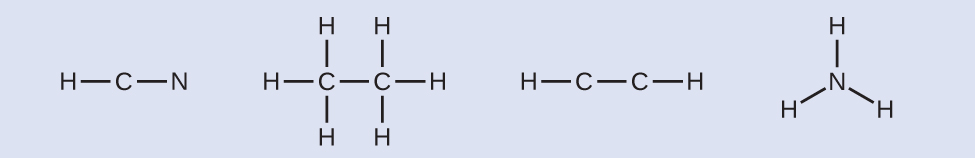3. Where needed, distribute electrons to the terminal atoms: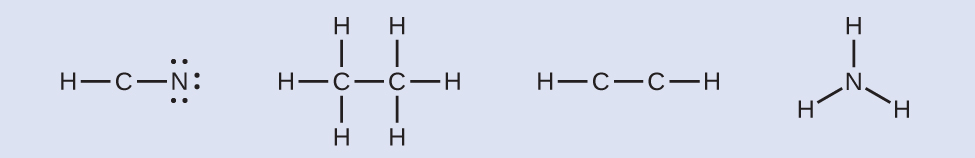HCN: six electrons placed on NH3CCH3: no electrons remainHCCH: no terminal atoms capable of accepting electronsNH3: no terminal atoms capable of accepting electrons
4. Where needed, place remaining electrons on the central atom: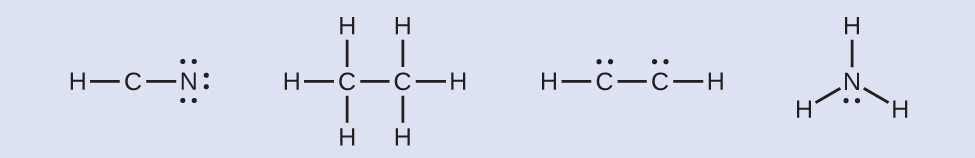HCN: no electrons remainH3CCH3: no electrons remainHCCH: four electrons placed on carbonNH3: two electrons placed on nitrogen
5. Where needed, rearrange electrons to form multiple bonds in order to obtain an octet on each atom:HCN: form two more C–N bondsH3CCH3: all atoms have the correct number of electronsHCCH: form a triple bond between the two carbon atomsNH3: all atoms have the correct number of electrons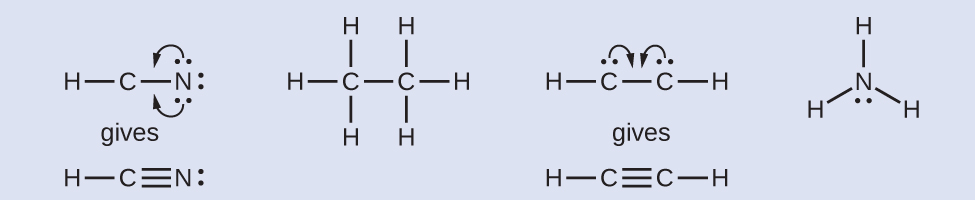Test yourself
Both carbon monoxide, CO, and carbon dioxide, CO2, are products of the combustion of fossil fuels. Both of these gases also cause problems: CO is toxic and CO2 has been implicated in global climate change. What are the Lewis structures of these two molecules?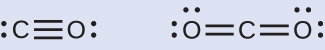### Example 4

What is the proper Lewis electron dot diagram for CO2?

Solution

The central atom is a C atom, with O atoms as surrounding atoms. We have a total of 4 + 6 + 6 = 16 valence electrons. Following the rules for Lewis electron dot diagrams for compounds gives us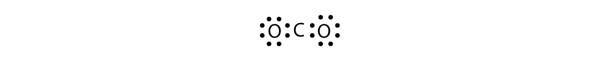The O atoms have complete octets around them, but the C atom has only four electrons around it. The way to solve this dilemma is to make a double bond between carbon and each O atom: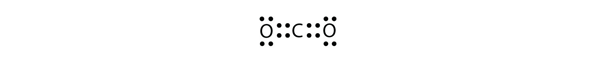Each O atom still has eight electrons around it, but now the C atom also has a complete octet. This is an acceptable Lewis electron dot diagram for CO2.

Test Yourself

What is the proper Lewis electron dot diagram for carbonyl sulfide (COS)?

### Fullerene Chemistry

Carbon soot has been known to man since prehistoric times, but it was not until fairly recently that the molecular structure of the main component of soot was discovered. In 1996, the Nobel Prize in Chemistry was awarded to Richard Smalley (Figure 1), Robert Curl, and Harold Kroto for their work in discovering a new form of carbon, the C60 buckminsterfullerene molecule (Figure 1 in Chapter 8 Introduction). An entire class of compounds, including spheres and tubes of various shapes, were discovered based on C60. This type of molecule, called a fullerene, shows promise in a variety of applications. Because of their size and shape, fullerenes can encapsulate other molecules, so they have shown potential in various applications from hydrogen storage to targeted drug delivery systems. They also possess unique electronic and optical properties that have been put to good use in solar powered devices and chemical sensors.Figure 1. Richard Smalley (1943–2005), a professor of physics, chemistry, and astronomy at Rice University, was one of the leading advocates for fullerene chemistry. Upon his death in 2005, the US Senate honored him as the “Father of Nanotechnology.” (credit: United States Department of Energy)

## Exceptions to the Octet Rule

Many covalent molecules have central atoms that do not have eight electrons in their Lewis structures. These molecules fall into three categories:

• Odd-electron molecules have an odd number of valence electrons, and therefore have an unpaired electron.
• Electron-deficient molecules have a central atom that has fewer electrons than needed for a noble gas configuration.
• Hypervalent molecules have a central atom that has more electrons than needed for a noble gas configuration.
Examples of these will be covered later chemistry courses.

### Food and Drink App: Vitamins and Minerals

Vitamins are nutrients that our bodies need in small amounts but cannot synthesize; therefore, they must be obtained from the diet. The word vitamin comes from “vital amine” because it was once thought that all these compounds had an amine group (NH2) in it. This is not actually true, but the name stuck anyway.

All vitamins are covalently bonded molecules. Most of them are commonly named with a letter, although all of them also have formal chemical names. Thus vitamin A is also called retinol, vitamin C is called ascorbic acid, and vitamin E is called tocopherol. There is no single vitamin B; there is a group of substances called the B complex vitamins that are all water soluble and participate in cell metabolism. If a diet is lacking in a vitamin, diseases such as scurvy or rickets develop. Luckily, all vitamins are available as supplements, so any dietary deficiency in a vitamin can be easily corrected.

A mineral is any chemical element other than carbon, hydrogen, oxygen, or nitrogen that is needed by the body. Minerals that the body needs in quantity include sodium, potassium, magnesium, calcium, phosphorus, sulfur, and chlorine. Essential minerals that the body needs in tiny quantities (so-called trace elements) include manganese, iron, cobalt, nickel, copper, zinc, molybdenum, selenium, and iodine. Minerals are also obtained from the diet. Interestingly, most minerals are consumed in ionic form, rather than as elements or from covalent molecules. Like vitamins, most minerals are available in pill form, so any deficiency can be compensated for by taking supplements.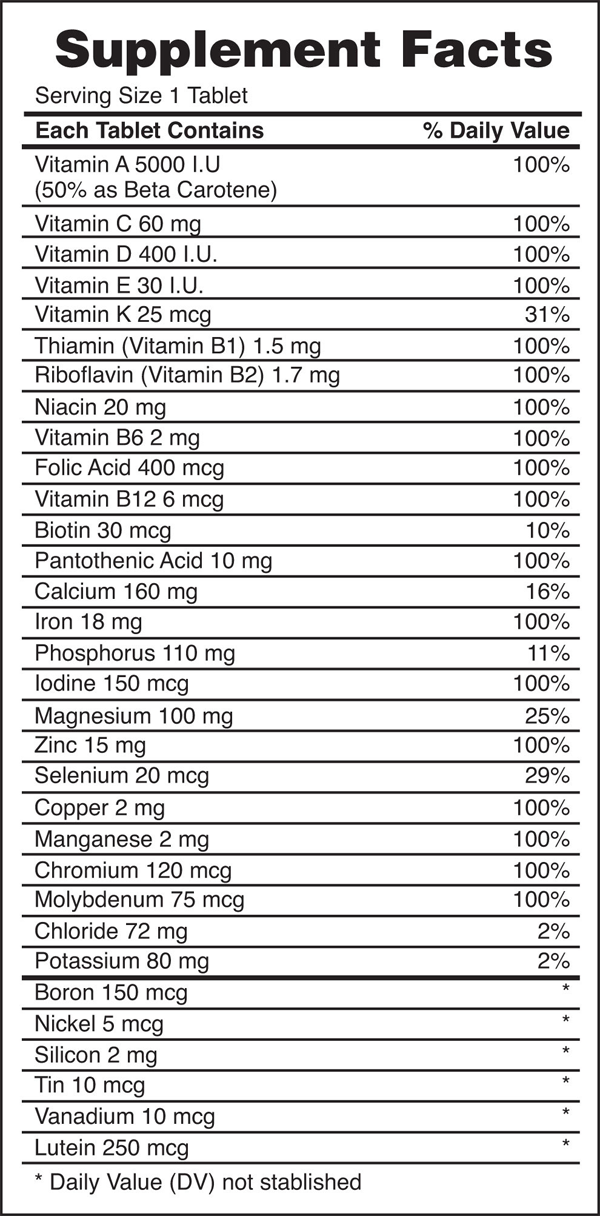Figure 2. Vitamin and Mineral Supplements

Every entry down through pantothenic acid is a vitamin, and everything from calcium and below is a mineral.

## Key Concepts and Summary

Valence electronic structures can be visualized by drawing Lewis symbols (for atoms and monatomic ions) and Lewis structures (for molecules and polyatomic ions). Lone pairs, unpaired electrons, and single, double, or triple bonds are used to indicate where the valence electrons are located around each atom in a Lewis structure. Most structures—especially those containing second row elements—obey the octet rule, in which every atom (except H) is surrounded by eight electrons. Exceptions to the octet rule occur for odd-electron molecules (free radicals), electron-deficient molecules, and hypervalent molecules.

### Exercises

1. Write the Lewis symbols for each of the following ions:

a) As3–         b) I–         c) Be2+         d) O2–          e) Ga3+          f) Li+         g) N3–

2. Write the Lewis symbols of the ions in each of the following ionic compounds and the Lewis symbols of the atom from which they are formed:

a) MgS         b) Al2O3            c) GaCl3          d) K2O        e) Li3N         f) KF

3. Write the Lewis structure for the diatomic molecule P2, an unstable form of phosphorus found in high-temperature phosphorus vapor.

4. Write Lewis structures for the following:

a) O2             b) H2CO         c) AsF3         d) ClNO         e) SiCl4

f) H3O+        g) NH4+            h) BF4−         i) HCCH         j) ClCN          k) C22+

5. Write Lewis structures for the following:

a)  SeCl3+          b) Cl2BBCl2 (contains a B–B bond)

6. Correct the following statement: “The bonds in solid PbCl2 are ionic; the bond in a HCl molecule is covalent. Thus, all of the valence electrons in PbCl2 are located on the Cl ions, and all of the valence electrons in a HCl molecule are shared between the H and Cl atoms.”

7. Methanol, H3COH, is used as the fuel in some race cars. Ethanol, C2H5OH, is used extensively as motor fuel in Brazil. Both methanol and ethanol produce CO2 and H2O when they burn. Write the chemical equations for these combustion reactions using Lewis structures instead of chemical formulas.

8. Carbon tetrachloride was formerly used in fire extinguishers for electrical fires. It is no longer used for this purpose because of the formation of the toxic gas phosgene, Cl2CO. Write the Lewis structures for carbon tetrachloride and phosgene.

9. The arrangement of atoms in several biologically important molecules is given here. Complete the Lewis structures of these molecules by adding multiple bonds and lone pairs. Do not add any more atoms.

a) the amino acid serine: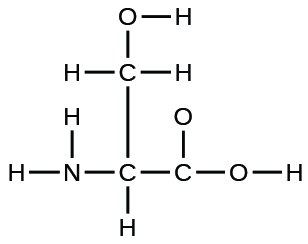b) urea: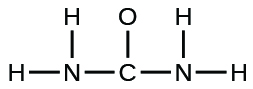c) pyruvic acid: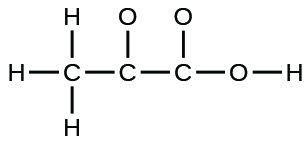d) uracil: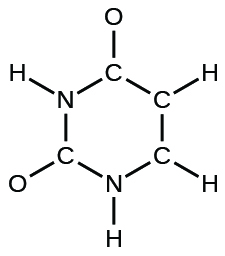e) carbonic acid: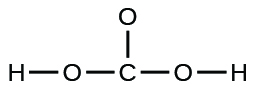10. A compound with a molar mass of about 42 g/mol contains 85.7% carbon and 14.3% hydrogen by mass. Write the Lewis structure for a molecule of the compound.

11. How are single, double, and triple bonds similar? How do they differ?

12. How many electrons will be in the valence shell of H atoms when it makes a covalent bond?

13. What is the Lewis electron dot diagram of I2? Circle the electrons around each atom to verify that each valence shell is filled.

14. What is the Lewis electron dot diagram of NCl3? Circle the electrons around each atom to verify that each valence shell is filled.

15. Draw the Lewis electron dot diagram for each substance.   a)  SF2         b)  BH4

16. Draw the Lewis electron dot diagram for each substance.   a)  GeH4     b)  ClF

17. Draw the Lewis electron dot diagram for each substance. Double or triple bonds may be needed.

a)  SiO2    b)  C2H4 (assume two central atoms)

18. Draw the Lewis electron dot diagram for each substance. Double or triple bonds may be needed.

a)  CS2       b)  NH2CONH2 (assume that the N and C atoms are the central atoms)

1. a) eight electrons: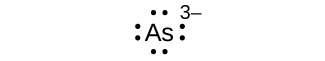b) eight electrons: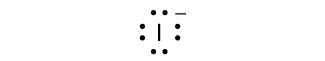c) no electrons:   Be2+

d) eight electrons: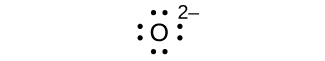e) no electrons:  Ga3+

f) no electrons: Li+

g) eight electrons: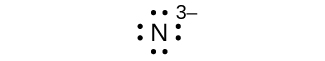2. a)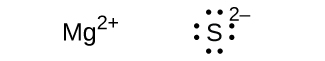b)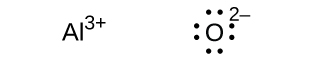c)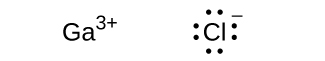d)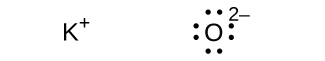e)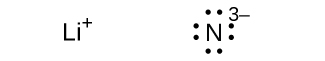f)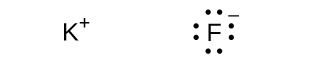3.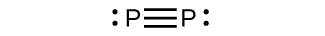4. a)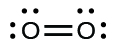In this case, the Lewis structure is inadequate to depict the fact that experimental studies have shown two unpaired electrons in each oxygen molecule.

b)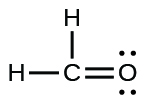c)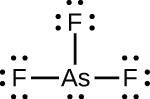d)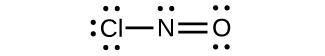e)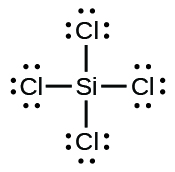f)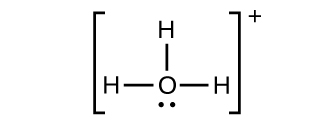g)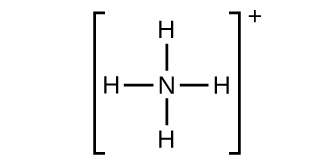h)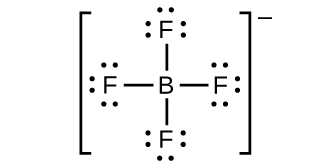i)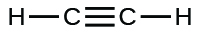j)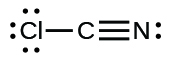k)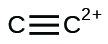5. a) SeCl3+: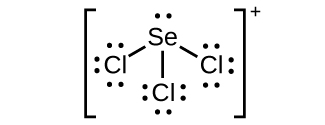b) Cl2BBCl2: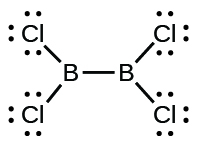6. Two valence electrons per Pb atom are transferred to Cl atoms; the resulting Pb2+ ion has a 6s2 valence shell configuration. Two of the valence electrons in the HCl molecule are shared, and the other six are located on the Cl atom as lone pairs of electrons.

7.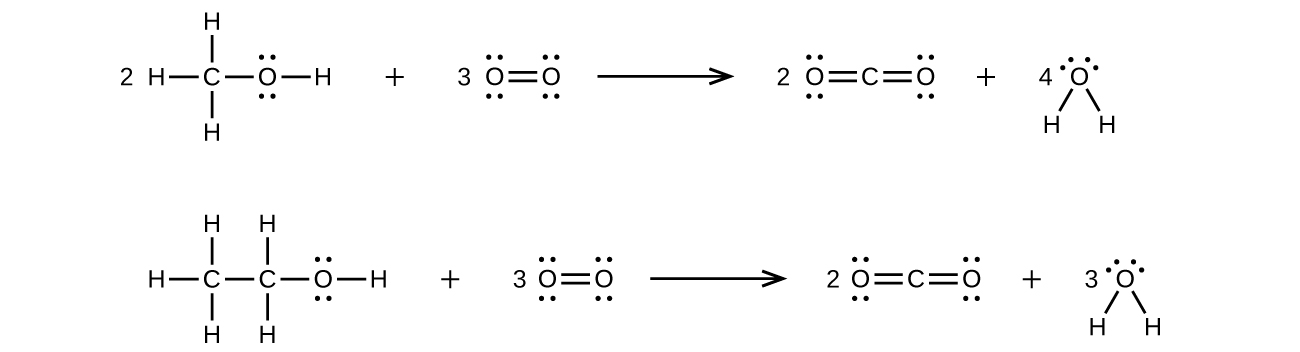8.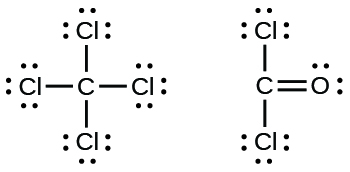9. a)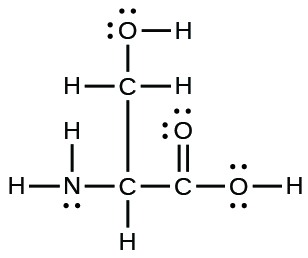b)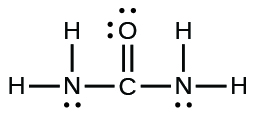c)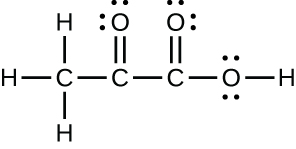d)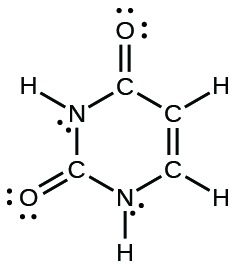e)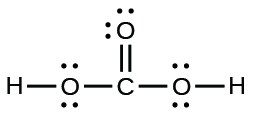10.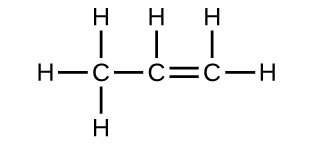11. Each bond includes a sharing of electrons between atoms. Two electrons are shared in a single bond; four electrons are shared in a double bond; and six electrons are shared in a triple bond.

12. two

13.14.15.

16.

17.

18.

## Glossary

double bond: covalent bond in which two pairs of electrons are shared between two atoms

free radical: molecule that contains an odd number of electrons

hypervalent molecule: molecule containing at least one main group element that has more than eight electrons in its valence shell

Lewis structure: diagram showing lone pairs and bonding pairs of electrons in a molecule or an ion

Lewis symbol: symbol for an element or monatomic ion that uses a dot to represent each valence electron in the element or ion

lone pair: two (a pair of) valence electrons that are not used to form a covalent bond

octet rule: guideline that states main group atoms will form structures in which eight valence electrons interact with each nucleus, counting bonding electrons as interacting with both atoms connected by the bond

single bond: bond in which a single pair of electrons is shared between two atoms

triple bond: bond in which three pairs of electrons are shared between two atoms